# DAV Class 4 Maths Chapter 10 Worksheet 2 Solutions

The DAV Maths Book Class 4 Solutions and DAV Class 4 Maths Chapter 10 Worksheet 2 Solutions of Angles offer comprehensive answers to textbook questions.

## DAV Class 4 Maths Ch 10 WS 2 Solutions

Question 1.
Write the name of the following angles.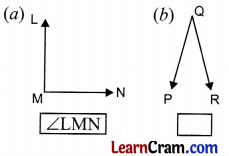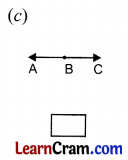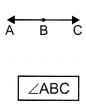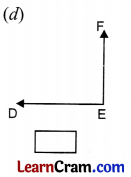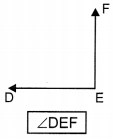Question 2.
You are given a few angles. Complete the table. The first one is done for you.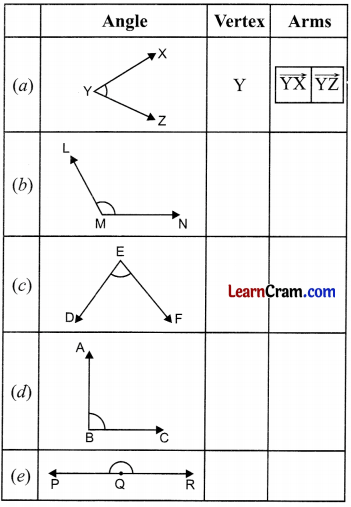Vertex Arms (b) M $$\overrightarrow{\mathrm{LM}}, \overrightarrow{\mathrm{MN}}$$ (c) E $$\overrightarrow{\mathrm{DE}}, \overrightarrow{\mathrm{EF}}$$ (d) B $$\overrightarrow{\mathrm{DE}}, \overrightarrow{\mathrm{EF}}$$ (e) Q $$\overrightarrow{\mathrm{PQ}}, \overrightarrow{\mathrm{QR}}$$

Question 3.
Draw the following angles.
(a) ∠MNP(b) ∠XYZ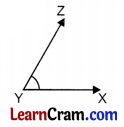(c) ∠BCD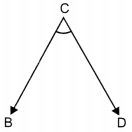Question 4.
How many angles are there in each figure?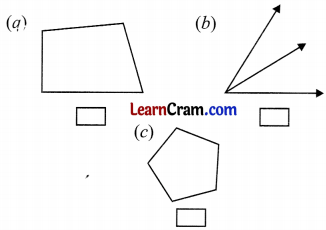(a) 4
(b) 3
(c) 5

### DAV Class 4 Maths Chapter 10 Worksheet 2 Notes

Vertex and Arms of Angles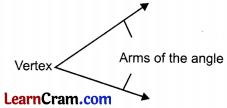• The common end point of two rays is called vertex of the angle.
• Letter denoting vertex should always be written in the middle.
• The two rays forming an angle are called the arms of the angle.